Enhance Readability of Code for Flow Charts

Appearance of Generated Code for Flow Charts

If you have Embedded Coder® and you generate code for models that include Stateflow® objects, the code from a flow chart resembles the samples that follow.

The following characteristics apply:

• By default, the generated code uses if-elseif-else statements to represent switch patterns. To convert the code to use switch-case statements, see Convert If-Elseif-Else Code to Switch-Case Statements.

• By default, variables that appear in the flow chart do not retain their names in the generated code. Modified identifiers make sure that no naming conflicts occur.

• Traceability comments for the transitions appear between each set of /* and */ markers. To learn more about traceability, see Trace Stateflow Elements in Generated Code.

Sample Code for a Decision Logic Pattern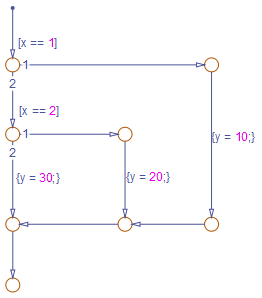if (modelname_U.In1 == 1.0) {
/* Transition: '<S1>:11' */
/* Transition: '<S1>:12' */
modelname_Y.Out1 = 10.0;

/* Transition: '<S1>:15' */
/* Transition: '<S1>:16' */
} else {
/* Transition: '<S1>:10' */
if (modelname_U.In1 == 2.0) {
/* Transition: '<S1>:13' */
/* Transition: '<S1>:14' */
modelname_Y.Out1 = 20.0;

/* Transition: '<S1>:16' */
} else {
/* Transition: '<S1>:17' */
modelname_Y.Out1 = 30.0;
}
}

Sample Code for an Iterative Loop Pattern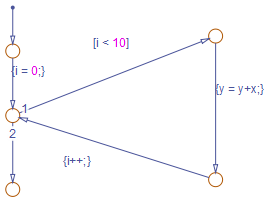for (sf_i = 0; sf_i < 10; sf_i++) {
/* Transition: '<S1>:40' */
/* Transition: '<S1>:41' */
modelname_B.y = modelname_B.y +
modelname_U.In1;

/* Transition: '<S1>:39' */
}

Sample Code for a Switch Pattern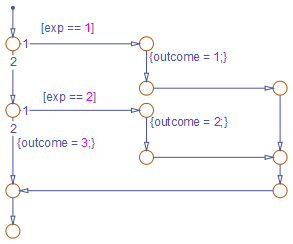if (modelname_U.In1 == 1.0) {
/* Transition: '<S1>:149' */
/* Transition: '<S1>:150' */
modelname_Y.Out1 = 1.0;

/* Transition: '<S1>:151' */
/* Transition: '<S1>:152' */
/* Transition: '<S1>:158' */
/* Transition: '<S1>:159' */
} else {
/* Transition: '<S1>:156' */
if (modelname_U.In1 == 2.0) {
/* Transition: '<S1>:153' */
/* Transition: '<S1>:154' */
modelname_Y.Out1 = 2.0;

/* Transition: '<S1>:155' */
/* Transition: '<S1>:158' */
/* Transition: '<S1>:159' */
} else {
/* Transition: '<S1>:161' */
modelname_Y.Out1 = 3.0;
}
}

Convert If-Elseif-Else Code to Switch-Case Statements

When you generate code for embedded real-time targets, you can choose to convert if-elseif-else code to switch-case statements. This conversion can enhance readability of the code. For example, when a flow chart contains a long list of conditions, the switch-case structure:

• Reduces the use of parentheses and braces

• Minimizes repetition in the generated code

How to Convert If-Elseif-Else Code to Switch-Case Statements

1

Verify that your flow chart follows the rules for conversion.

Verify the Contents of the Flow Chart
2

Enable the conversion and generate code for your model.

Enable the Conversion
3

Troubleshoot the generated code.

• If you see switch-case statements for your flow chart, you can stop.

• If you see if-elseif-else statements for your flow chart, update the chart and repeat the previous step.

Troubleshoot the Generated Code

Rules of Conversion

For the conversion to occur, following these guidelines. LHS and RHS refer to the left-hand side and right-hand side of a condition, respectively.

ConstructRules to Follow
Flow chart

Must have a default and two or more unique conditions.

Each condition

Must test equality only.

Must use the same variable or expression for the LHS. You can reverse the LHS and RHS.

Each LHS

Must be a single variable or expression.

Cannot be a constant.

Must have an integer or enumerated data type.

Cannot have any side effects on simulation.

For example, the LHS can read from but not write to global variables.

Each RHS

Must be a constant or a parameter.

Must have an integer or enumerated data type.

Duplicate Conditions

If a flow chart has duplicate conditions, the conversion preserves only the first condition. The code discards the other instances of duplicate conditions.

After removal of duplicates, two or more unique conditions must exist. If not, the conversion does not occur and the code contains all duplicate conditions.

Example of Generated CodeCode After Conversion
if (x == 1) {
block1
} else if (x == 2) {
block2
} else if (x == 1) {  // duplicate
block3
} else if (x == 3) {
block4
} else if (x == 1) {  // duplicate
block5
} else {
block6
}
switch (x) {
case 1:
block1; break;
case 2:
block2; break;
case 3:
block4; break;
default:
block6; break;
}
if (x == 1) {
block1
} else if (x == 1) {  // duplicate
block2
} else {
block3
}

No change, because only one unique condition exists

Example of Converting Code to Switch-Case Statements

Suppose that you have this model containing a single chart.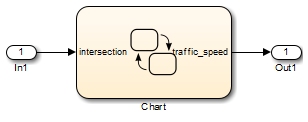The chart contains a flow chart and four MATLAB® functions.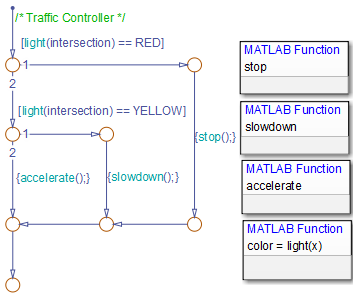The MATLAB functions in the chart contain the code listed in the table. In each case, the Function Inline Option is Auto. For more information about function inlining, see Specify Properties of Graphical Functions (Stateflow).

MATLAB FunctionCode
stop
function stop
%#codegen
coder.extrinsic('disp');
disp('Not moving.')

traffic_speed = 0;
slowdown
function slowdown
%#codegen
coder.extrinsic('disp')
disp('Slowing down.')

traffic_speed = 1;
accelerate
function accelerate
%#codegen
coder.extrinsic('disp');
disp('Moving along.')

traffic_speed = 2;
light
function color = light(x)
%#codegen
if (x < 20)
color = TrafficLights.GREEN;
elseif (x >= 20 && x < 25)
color = TrafficLights.YELLOW;
else
color = TrafficLights.RED;
end

The output color of the function light uses the enumerated type TrafficLights. The enumerated type definition in TrafficLights.m is:

enumeration
RED(0)
YELLOW(5)
GREEN(10)
end
end

Verify the Contents of the Flow Chart

Check that the flow chart in your Stateflow chart follows the rules in Rules of Conversion.

ConstructHow the Construct Follows the Rules
Flow chart

A default condition and two unique conditions exist:

• [light(intersection) == RED]

• [light(intersection) == YELLOW]

Each condition

Each condition:

• Tests equality

• Uses the same function call light(intersection) for the LHS

Each LHS

Each LHS:

• Contains a single expression

• Is the output of a function call, not a constant

• Is of enumerated type TrafficLights, which you define in TrafficLights.m on the MATLAB path. See Define Enumerated Data Types (Stateflow).

• Uses a function call that does not have side effects

Each RHS

Each RHS:

• Is an enumerated value and therefore a constant

• Is of enumerated type TrafficLights

Enable the Conversion

1. Open the Model Configuration Parameters dialog box.

2. In the Code Generation pane, select ert.tlc for the System target file for an ERT-based target for your model.

3. In the Code Generation > Code Style pane, select the Convert if-elseif-else patterns to switch-case statements check box.

If you select this check box, the conversion applies to:

• Flow charts in all charts of a model

• MATLAB functions in all charts of a model

• All MATLAB Function blocks in that model

4. In the model, on the C Code tab, click to generate source code from the model.

Troubleshoot the Generated Code

The generated code for the flow chart appears like this code:

if (sf_color == RED) {
/* Transition: '<S1>:11' */
/* Transition: '<S1>:12' */
/* MATLAB Function 'stop': '<S1>:23' */
/* '<S1>:23:6' */
rtb_traffic_speed = 0;

/* Transition: '<S1>:15' */
/* Transition: '<S1>:16' */
} else {
/* Transition: '<S1>:10' */
/* MATLAB Function 'light': '<S1>:19' */
if (ifelse_using_enums_U.In1 < 20.0) {
/* '<S1>:19:3' */
/* '<S1>:19:4' */
sf_color = GREEN;
} else if ((ifelse_using_enums_U.In1 >= 20.0) &&
(ifelse_using_enums_U.In1 < 25.0)) {
/* '<S1>:19:5' */
/* '<S1>:19:6' */
sf_color = YELLOW;
} else {
/* '<S1>:19:8' */
sf_color = RED;
}

if (sf_color == YELLOW) {
/* Transition: '<S1>:13' */
/* Transition: '<S1>:14' */
/* MATLAB Function 'slowdown': '<S1>:24' */
/* '<S1>:24:6' */
rtb_traffic_speed = 1;

/* Transition: '<S1>:16' */
} else {
/* Transition: '<S1>:17' */
/* MATLAB Function 'accelerate': '<S1>:25' */
/* '<S1>:25:6' */
rtb_traffic_speed = 2;
}
}

Because the MATLAB function light appears inlined, inequality comparisons appear in these lines of code:

if (ifelse_using_enums_U.In1 < 20.0) {
....
} else if ((ifelse_using_enums_U.In1 >= 20.0) &&
(ifelse_using_enums_U.In1 < 25.0)) {
....

Because inequalities appear in the body of the if-elseif-else code for the flow chart, the conversion to switch-case statements does not occur. Do one of the following:

Change the Inlining Property for the Function.  If you do not want to modify your flow chart, change the inlining property for the function light:

1. Right-click the function box for light and select Properties.

2. In the properties dialog box, for Function Inline Option, select Function.

3. Click to close the dialog box.

Note

You do not have to change the inlining property for the other three MATLAB functions in the chart. Because the flow chart does not call those functions during evaluation of conditions, the inlining property for those functions can remain Auto.

When you regenerate code for your model, the code for the flow chart now appears like this code:

switch (ifelse_using_enums_light(ifelse_using_enums_U.In1)) {
case RED:
/* Transition: '<S1>:11' */
/* Transition: '<S1>:12' */
/* MATLAB Function 'stop': '<S1>:23' */
/* '<S1>:23:6' */
ifelse_using_enums_Y.Out1 = 0.0;

/* Transition: '<S1>:15' */
/* Transition: '<S1>:16' */
break;

case YELLOW:
/* Transition: '<S1>:10' */
/* Transition: '<S1>:13' */
/* Transition: '<S1>:14' */
/* MATLAB Function 'slowdown': '<S1>:24' */
/* '<S1>:24:6' */
ifelse_using_enums_Y.Out1 = 1.0;

/* Transition: '<S1>:16' */
break;

default:
/* Transition: '<S1>:17' */
/* MATLAB Function 'accelerate': '<S1>:25' */
/* '<S1>:25:6' */
ifelse_using_enums_Y.Out1 = 2.0;
break;
}

Because the MATLAB function light no longer appears inlined, the conversion to switch-case statements occurs. To enhance readability, the switch-case statements provide these benefits:

• The code reduces the use of parentheses and braces.

• The LHS expression ifelse_using_enums_light(ifelse_using_enums_U.In1) appears only once, minimizing repetition in the code.

Modify the Flow Chart to Ensure Switch-Case Statements.  If you do not want to change the inlining property for the function light, modify your flow chart:

1. Add chart local data color_out with the enumerated type TrafficLights.

2. Replace each instance of light(intersection) with color_out.

3. Add the action {color_out = light(intersection)} to the default transition of the flow chart.

The chart now looks similar to this chart: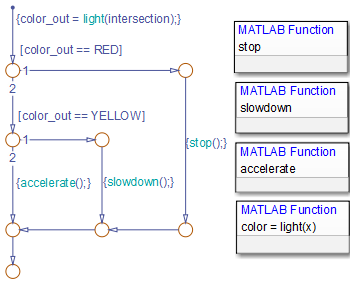When you regenerate code for your model, the code for the flow chart uses switch-case statements.